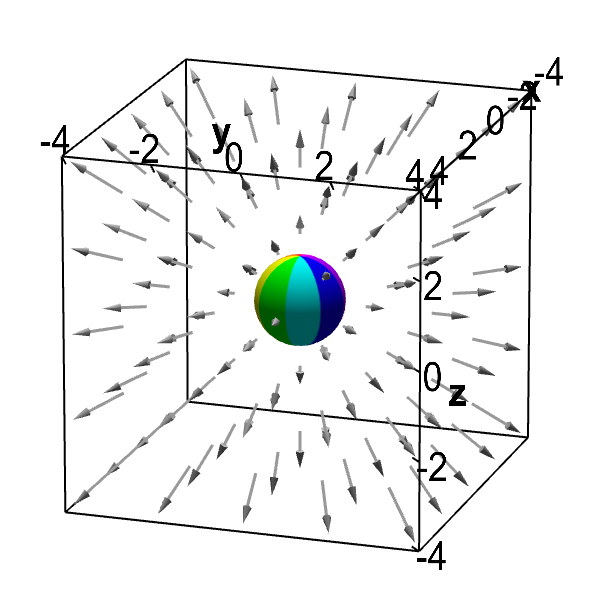# Math Insight

### Applet: Divergent vector field with embedded sphereThe sphere embedded in this vector field can be used to visualize the divergence of this vector field. If the vector field $\dlvf(x,y,z)$ indicates the velocity of a fluid at point $(x,y,z)$, then one can visualize how much fluid is flowing in and out of the sphere by the direction and length of the vectors.

When the sphere is at the origin, it is clear that fluid is moving out of the sphere. When the sphere is moved away from the origin, fluid flows into the side of the sphere closest to the origin, and fluid flows out of the side of the sphere further from the origin. Since the fluid speeds up as it gets further from the origin (indicated by the increasing length of the vectors), the fluid is flowing out of the sphere at a greater velocity than it is flowing in. In addition, since the velocity field is radiating outward, fluid is flowing out of the sphere over more than half of its surface. This visualization suggests that more fluid is flowing out of the sphere than is flowing into the sphere, no matter the sphere's position. Hence, it appears the fluid may be expanding.

Indeed, the vector field does have a positive divergence. The vector field is $\dlvf(x,y,z) = (x,y,z)$. Its divergence is $\pdiff{\dlvfc_1}{x}+\pdiff{\dlvfc_2}{y}+\pdiff{\dlvfc_3}{z}=1+1+1=3$. The vector field has a constant, positive divergence everywhere.